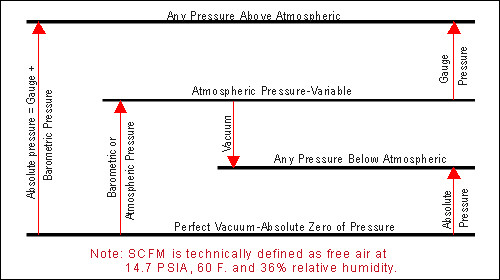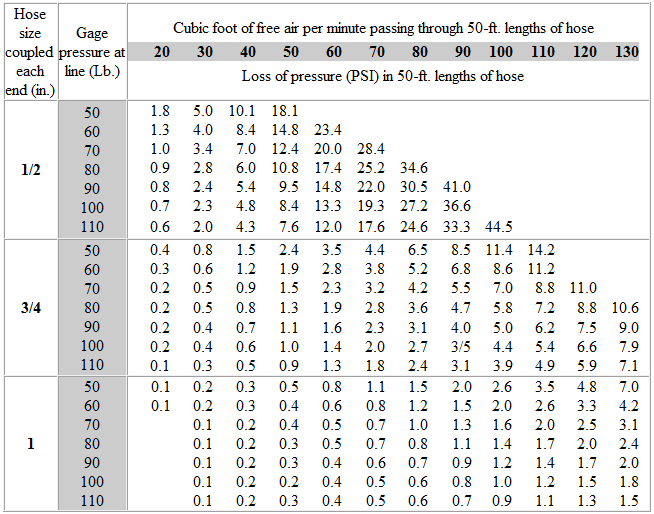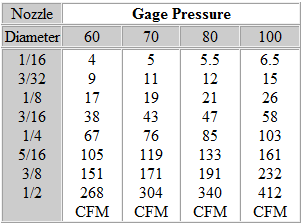# Pressure Converter

 From: To: pascal [Pa]exapascal [EPa]petapascal [PPa]terapascal [TPa]gigapascal [GPa]megapascal [MPa]kilopascal [kPa]hectopascal [hPa]dekapascal [daPa]decipascal [dPa]centipascal [cPa]millipascal [mPa]micropascal [µPa]nanopascal [nPa]picopascal [pPa]femtopascal [fPa]attopascal [aPa]newton/square meter [N/m^2]newton/square centimeternewton/square millimeterkilonewton/square meterbarmillibar [mbar]microbar [µbar]dyne/square centimeterkilogram-force/square meterkilogram-force/sq. centimeterkilogram-force/sq. millimetergram-force/square centimeterton-force (short)/square footton-force (short)/square inchton-force (long)/square footton-force (long)/square inchkip-force/square inch [kipf/in^2]ksi [ksi]pound-force/square footpound-force/square inchpsi [psi]poundal/square foot [pdl/ft^2]torr [Torr]centimeter mercury (0°C)millimeter mercury (0°C)inch mercury (32°F) [inHg]inch mercury (60°F) [inHg]centimeter water (4°C) [cmAq]millimeter water (4°C) [mmAq]inch water (4°C) [inAq]foot water (4°C) [ftAq]inch water (60°F) [inAq]foot water (60°F) [ftAq]atmosphere technical [at]Standard atmosphere [atm] pascal [Pa]exapascal [EPa]petapascal [PPa]terapascal [TPa]gigapascal [GPa]megapascal [MPa]kilopascal [kPa]hectopascal [hPa]dekapascal [daPa]decipascal [dPa]centipascal [cPa]millipascal [mPa]micropascal [µPa]nanopascal [nPa]picopascal [pPa]femtopascal [fPa]attopascal [aPa]newton/square meter [N/m^2]newton/square centimeternewton/square millimeterkilonewton/square meterbarmillibar [mbar]microbar [µbar]dyne/square centimeterkilogram-force/square meterkilogram-force/sq. centimeterkilogram-force/sq. millimetergram-force/square centimeterton-force (short)/square footton-force (short)/square inchton-force (long)/square footton-force (long)/square inchkip-force/square inch [kipf/in^2]ksi [ksi]pound-force/square footpound-force/square inchpsi [psi]poundal/square foot [pdl/ft^2]torr [Torr]centimeter mercury (0°C)millimeter mercury (0°C)inch mercury (32°F) [inHg]inch mercury (60°F) [inHg]centimeter water (4°C) [cmAq]millimeter water (4°C) [mmAq]inch water (4°C) [inAq]foot water (4°C) [ftAq]inch water (60°F) [inAq]foot water (60°F) [ftAq]atmosphere technical [at]Standard atmosphere [atm] Result:

# Flow Converter

 From: To: cubic meter/second [m^3/s]cubic meter/day [m^3/d]cubic meter/hour [m^3/h]cubic meter/minute [m^3/min]cubic centimeter/day [cm^3/d]cubic centimeter/hour [cm^3/h]cubic centimeter/minutecubic centimeter/secondliter/day [L/d]liter/hour [L/h]liter/minute [L/min]liter/second [L/s]milliliter/day [mL/d]milliliter/hour [mL/h]milliliter/minute [mL/min]milliliter/second [mL/s]gallon (US)/day [gal (US)/d]gallon (US)/hour [gal (US)/h]gallon (US)/minutegallon (US)/second [gal (US)/s]gallon (UK)/day [gal (UK)/d]gallon (UK)/hour [gal (UK)/h]gallon (UK)/minutegallon (UK)/second [gal (UK)/s]kilobarrel (US)/daybarrel (US)/day [bbl (US)/d]barrel (US)/hour [bbl (US)/h]barrel (US)/minutebarrel (US)/second [bbl (US)/s]acre-foot/year [ac*ft/y]acre-foot/day [ac*ft/d]acre-foot/hour [ac*ft/h]hundred-cubic foot/dayhundred-cubic foot/hourhundred-cubic foot/minuteounce/hour [oz/h]ounce/minute [oz/min]ounce/second [oz/s]ounce (UK)/hour [oz (UK)/h]ounce (UK)/minuteounce (UK)/second [oz (UK)/s]cubic yard/hour [yd^3/h]cubic yard/minute [yd^3/min]cubic yard/second [yd^3/s]cubic foot/hour [ft^3/h]cubic foot/minute [ft^3/min]cubic foot/second [ft^3/s]cubic inch/hour [in^3/h]cubic inch/minute [in^3/min]cubic inch/second [in^3/s] cubic meter/second [m^3/s]cubic meter/day [m^3/d]cubic meter/hour [m^3/h]cubic meter/minute [m^3/min]cubic centimeter/day [cm^3/d]cubic centimeter/hour [cm^3/h]cubic centimeter/minutecubic centimeter/secondliter/day [L/d]liter/hour [L/h]liter/minute [L/min]liter/second [L/s]milliliter/day [mL/d]milliliter/hour [mL/h]milliliter/minute [mL/min]milliliter/second [mL/s]gallon (US)/day [gal (US)/d]gallon (US)/hour [gal (US)/h]gallon (US)/minutegallon (US)/second [gal (US)/s]gallon (UK)/day [gal (UK)/d]gallon (UK)/hour [gal (UK)/h]gallon (UK)/minutegallon (UK)/second [gal (UK)/s]kilobarrel (US)/daybarrel (US)/day [bbl (US)/d]barrel (US)/hour [bbl (US)/h]barrel (US)/minutebarrel (US)/second [bbl (US)/s]acre-foot/year [ac*ft/y]acre-foot/day [ac*ft/d]acre-foot/hour [ac*ft/h]hundred-cubic foot/dayhundred-cubic foot/hourhundred-cubic foot/minuteounce/hour [oz/h]ounce/minute [oz/min]ounce/second [oz/s]ounce (UK)/hour [oz (UK)/h]ounce (UK)/minuteounce (UK)/second [oz (UK)/s]cubic yard/hour [yd^3/h]cubic yard/minute [yd^3/min]cubic yard/second [yd^3/s]cubic foot/hour [ft^3/h]cubic foot/minute [ft^3/min]cubic foot/second [ft^3/s]cubic inch/hour [in^3/h]cubic inch/minute [in^3/min]cubic inch/second [in^3/s] Result:

# Temperature Converter

 From: To: kelvin [K]degree Celsius [°C]degree Fahrenheit [°F]degree Rankine [°R]degree Reaumur [°r]Triple point of water kelvin [K]degree Celsius [°C]degree Fahrenheit [°F]degree Rankine [°R]degree Reaumur [°r]Triple point of water Result:

# Air Moisture Content

Grains of water in one cubic foot of air at various temperatures and percentages of saturation. On average, for every 20 degrees Fahrenheit temperature drop, we loose one half the water.# Dew Point Conversion Chart# Expanding Pressure# Cost of Compressed Air

15% Fixed Charges: Compressor, Installation, Depreciation, Insurance, Repair.
15% Operating Charges: Attendance, Lubrication, Cooling-Water, Filters.
70% Power: Electric Motor Driven (both energy & demand) at 6 cents/kW hr.

20 cents per 1000 CF @ 100 PSIG: 200 HP = 1000 CFM X 60 min. X 24 hr. = \$288.00 per day.
Rule of Thumb: Cost \$1.00 per HP per 24 hrs. at 6 cents per kW hour.
Cost of Air Leakage 1/4″ leak = 2,920,000 cu. ft. per month = \$684.00.

# Discharge, Cubic Feet, Free Air Per Minute# Friction of Air in Hose# Pipe Size Recommendation (80-125 PSI)# Sand Blasting Nozzles